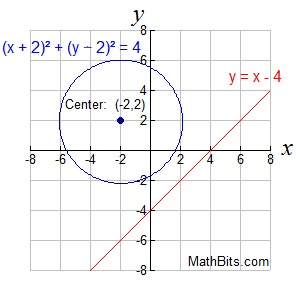What Do Linear Quadratic Systems Look Like? MathBitsNotebook.com Terms of Use   Contact Person: Donna Roberts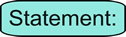When you are working with quadratics, you are primarily working with ax2 + bx + c = 0  or  y = ax2 + bx + c (where a, b and c are constants).

A linear quadratic system is a system containing one linear equation and one quadratic equation
(which is generally one straight line and one parabola).
A simple linear system contains two linear equations (which is two straight lines).

When dealing with a straight line and a parabola, there are three possible ways they
may appear on a graph, giving three possible solution situations.Possible Solution Situations Linear-Quadratic System (line and parabola) A solution is a location where the straight line and the parabola intersect (cross).

 Situation 1: When graphed, most linear quadratic systems will show the line and the parabola intersecting in two points, as seen at the right. Two solutions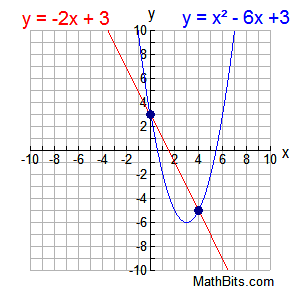Situation 2: If the straight line is tangent to the parabola, it will intersect (hit) the parabola in only one location, as seen at the right. One solution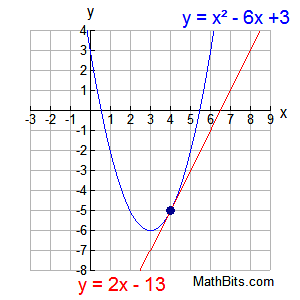Situation 3: It is possible that the straight line and the parabola never touch one another. They do not intersect. No solutions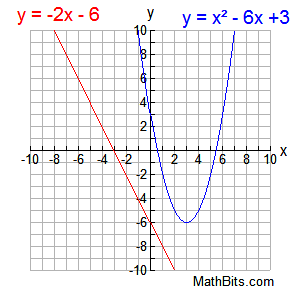It is also possible when working with quadratics, that you may encounter a quadratic where both the x and y variables are squared (with the same coefficients); a circle.

When dealing with a straight line and a circle, there are also three possible ways they
may appear on a graph, giving three possible solution situations.Possible Solution Situations Linear-Quadratic System (line and circle) A solution is a location where the straight line and the circle intersect (cross).
 Situation 1: When graphed, most linear quadratic systems will show the line and the circle intersecting in two points, as seen at the right. Two solutions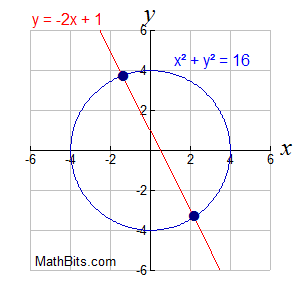Situation 2: If the straight line is tangent to the circle, it will intersect (hit) the circle in only one location, as seen at the right. One solution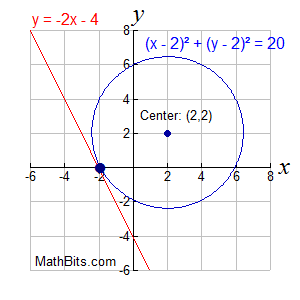Situation 3: It is possible that the straight line and the circle never touch one another. They do not intersect. No solutions